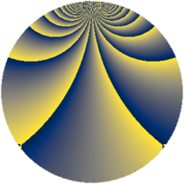# Properties

 Label 552.2.bLevel $552$ Weight $2$ Character orbit 552.b Rep. character $\chi_{552}(413,\cdot)$ Character field $\Q$ Dimension $92$ Newform subspaces $3$ Sturm bound $192$ Trace bound $6$

# Related objects

## Defining parameters

 Level: $$N$$ $$=$$ $$552 = 2^{3} \cdot 3 \cdot 23$$ Weight: $$k$$ $$=$$ $$2$$ Character orbit: $$[\chi]$$ $$=$$ 552.b (of order $$2$$ and degree $$1$$) Character conductor: $$\operatorname{cond}(\chi)$$ $$=$$ $$552$$ Character field: $$\Q$$ Newform subspaces: $$3$$ Sturm bound: $$192$$ Trace bound: $$6$$ Distinguishing $$T_p$$: $$5$$, $$29$$

## Dimensions

The following table gives the dimensions of various subspaces of $$M_{2}(552, [\chi])$$.

Total New Old
Modular forms 100 100 0
Cusp forms 92 92 0
Eisenstein series 8 8 0

## Trace form

 $$92q - 9q^{6} - 4q^{9} + O(q^{10})$$ $$92q - 9q^{6} - 4q^{9} + q^{12} + 8q^{16} - q^{18} - 8q^{24} - 84q^{25} - 24q^{31} + 7q^{36} + 20q^{39} - 24q^{46} + 53q^{48} - 76q^{49} - 42q^{52} - 32q^{54} + 32q^{55} - 10q^{58} + 6q^{64} + 72q^{70} + 68q^{72} - 8q^{73} + 13q^{78} + 12q^{81} + 18q^{82} - 4q^{87} - 66q^{94} + 11q^{96} + O(q^{100})$$

## Decomposition of $$S_{2}^{\mathrm{new}}(552, [\chi])$$ into newform subspaces

Label Dim. $$A$$ Field CM Traces $q$-expansion
$$a_2$$ $$a_3$$ $$a_5$$ $$a_7$$
552.2.b.a $$6$$ $$4.408$$ 6.0.8869743.1 $$\Q(\sqrt{-23})$$ $$0$$ $$0$$ $$0$$ $$0$$ $$q+\beta _{1}q^{2}+\beta _{4}q^{3}+(\beta _{2}+\beta _{5})q^{4}+(\beta _{2}+\cdots)q^{6}+\cdots$$
552.2.b.b $$6$$ $$4.408$$ 6.0.8869743.1 $$\Q(\sqrt{-23})$$ $$0$$ $$0$$ $$0$$ $$0$$ $$q-\beta _{1}q^{2}+\beta _{2}q^{3}+(\beta _{2}+\beta _{5})q^{4}+(1+\cdots)q^{6}+\cdots$$
552.2.b.c $$80$$ $$4.408$$ None $$0$$ $$0$$ $$0$$ $$0$$